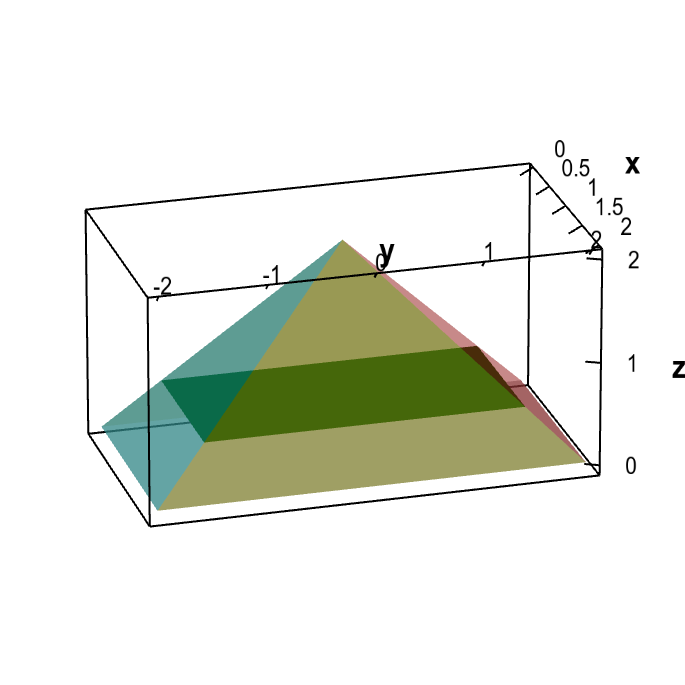# Math Insight

### Applet: Demonstrating the cross section method for computing triple integral limitsThe transparent region is a pyramid bounded by the planes $z=0$, $z=4-2x$, $z=2-y$, $z=2x$, and $z=2+y$. The cross sections perpendicular to the $z$-axis are rectangles, as illustrated by the single green cross section shown. By moving the rectangle up or down, you can change the value of $z$ at which the cross section is calculated.